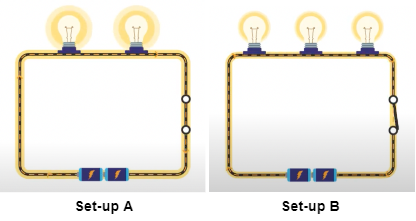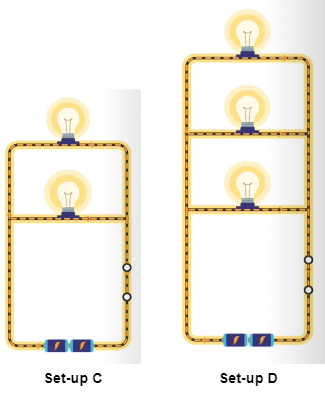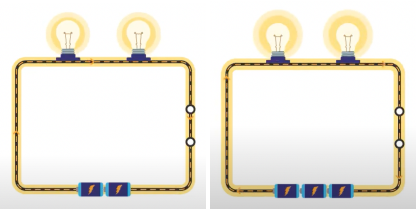Study P5 Science Systems: Electrical Systems - Geniebook# Systems: Electrical Systems

In this article, we are going to learn about Electrical Systems according to the Singapore Primary 5 Science syllabus. We will examine various concepts like open and closed circuits, as well as factors affecting the brightness of bulbs in an electrical circuit.

This article contains concepts that will definitely be helpful when you are answering questions that require you to determine if a certain light bulb will light up, or how the brightness of the bulbs will be affected when certain components are added to the circuit.

## What is an Electrical Circuit?

An electrical circuit is an electrical system that is made up of electrical components (such as batteries, bulbs and wires) which will work together when the circuit is closed.

### Closed Circuit

An electric current flows through the circuit when the bulb, battery and wire are properly connected together. This is known as a closed circuit, where all parts are properly connected to each other and there are no gaps between the parts.

### Open Circuit

In an open circuit, not all parts are properly connected together. For example, the positive terminal of a battery may be connected to the positive terminal of another battery, which results in electric current not being able to flow through the circuit.

Another factor resulting in an open circuit will be the presence of one or more gaps between the electrical components. For example, a switch in the “open” position will result in a gap within the circuit.

### Brightness Of Bulbs

The brightness of a bulb depends on the amount of electric current flowing through each bulb in a circuit. We will now examine how the following factors affect the brightness of the bulbs:

1. Number of bulbs arranged in series
2. Number of bulbs arranged in parallel
3. Number of batteries arranged in series

Series Arrangement: When electrical components are connected one after another.

Parallel Arrangement: When electrical components are connected parallel to each other.

1. Number Of Bulbs Arranged In Series

When more bulbs are added in series, the amount of electric current passing through each bulb decreases. Hence, the brightness of each bulb decreases.

As we can see from the following diagrams, each of the two bulbs arranged in series in Set-up A is brighter than each of the three bulbs arranged in series in Set-up B. Adding a light bulb in series arrangement decreases the brightness of the bulbs.1. Number Of Bulbs Arranged In Parallel

When more bulbs are added in parallel, the amount of electric current passing through each bulb remains the same. Hence, the brightness of each bulb remains the same.

As we can see from the following diagrams, each of the three bulbs arranged in parallel in Set-up D is as bright as each of the two bulbs arranged in parallel in Set-up C. Adding a bulb in parallel arrangement has no effect on the brightness of the bulbs.1. Number Of Batteries Arranged In Series

When more batteries are added in series, the amount of electric current passing through each bulb increases. Hence, the brightness of each bulb increases.

As we can see from the following diagrams, each of the two bulbs in Set-up F (with three batteries connected) is brighter than each of the two bulbs in Set-up E (with two batteries connected). Adding a battery (in series) causes the brightness of the bulbs to increase.Set-up E                                             Set-up F

## Conclusion

In this article, we have learned about the various types of electrical circuits - open or closed.

We have also examined how bulbs and batteries can be arranged in series and parallel, and how the various arrangements can affect the brightness of bulbs.

I hope you have learnt a lot from reading this article and will continue to practise on Science questions that are related to Electrical Systems so that you will excel in this topic!

Answer the following questions based on the concepts we’ve covered in this article. If you are unclear, you may want to revisit the relevant section to revise the concepts.

Question 1:

In the circuit given below, which of the switches should be left open and which should be closed so that only Bulb A lights up?Switch W Switch X Switch Y Switch Z ( 1 ) Closed Closed Open Open ( 2 ) Open Closed Closed Closed ( 3 ) Closed Closed Open Closed ( 4 ) Open Closed Open Closed

Solution:

(3)

Explanation:

For electricity to flow in a closed path through bulb A, switches W, X and Z must be closed.

Since we do not want Bulb B to light up, we will keep Switch Y open so that no current flows in the branch with Bulb B.

Question 2:

Nicky was given two circuits as shown below. All the bulbs and the batteries used are the same and are in good working condition.In which circuit will the bulbs light up more brightly? Explain your answer.

Solution:

Circuit A

Explanation:

There are fewer bulbs arranged in series in Circuit A. Hence, a larger current flows through the bulbs in Circuit A than those in Circuit B, causing them to light up more brightly.

Question 3:

With reference to the diagram given below, list down the disadvantage of arranging the bulbs as given in Circuit A and Circuit B.Solution:

Both Circuits A and B have the bulbs in a series arrangement.

When one of the bulbs connected in the series circuit fuses, the other bulbs also will not light up because there is a gap in the circuit, creating an open circuit.

Question 4a:

The graph below shows the number of batteries, up to five batteries in a circuit, and the brightness of the bulb in the circuit.In what arrangement were the first four batteries added to the circuit?

Solution:

The first four batteries were added to the circuit in series.

Question 4b:Why was the brightness of the bulb zero when the fifth battery was added?

Solution:

There will be excess electric current flowing through the light bulb, causing it to fuse. As the light bulb is fused, it will not light up and there will be zero brightness.

Continue Learning
Systems: Electrical Systems Plant Reproduction: Structures And Pollination
Human Reproduction: Parts And FunctionsPrimaryPrimary 1Primary 2Primary 3Primary 4Primary 5EnglishMathsScience
Systems: Electrical Systems
Plant Reproduction: Structures And Pollination
Human Reproduction: Parts And FunctionsPrimary 6SecondarySecondary 1Secondary 2Secondary 3Secondary 4